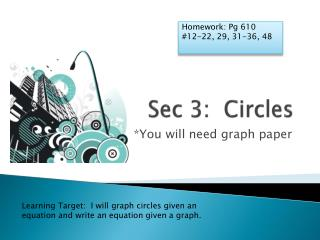DownloadDownload PresentationSec 3: Circles

Sec 3: Circles

Télécharger la présentationSec 3: Circles

- - - - - - - - - - - - - - - - - - - - - - - - - - - E N D - - - - - - - - - - - - - - - - - - - - - - - - - - -
Presentation Transcript

1. Homework: Pg610 #12-22, 29, 31-36, 48 Sec 3: Circles *You will need graph paper Learning Target: I will graph circles given an equation and write an equation given a graph.

3. Standard Form of an Equation of a Circle • The standard form of an equation of a circle with center (h, k) and radius r is

4. Ex 1: Write the equation for the following circles. • Center: (-4, 3) and radius = 4. • Center: (0, -2) and radius of 11.

5. Ex 1 Continued C. D.

6. Ex 2: Graphing Circles Given an Equation • Graph (x – 16)² + (y + 9)² = 144 Center: Radius = • Domain: • Range: (16, -9) √144 = 12 4 ≤ x ≤ 28 -21 ≤ y ≤ 3

7. Ex 3: State the Center, Radius, Domain and Range and then Graph the circle. • (x + 8)² + (y + 3)² = 121 • x² + y² = 10 Center: (-8, -3) r = 11 D: -19 ≤ x ≤ 3 R: -14 ≤ y ≤ 8 Center: (0, 0) r = √10 or 3.2 D: -3.2 ≤ x ≤ 3.2 R: -3.2 ≤ y ≤ 3.2

8. Ex 4: This is Trickery! • Tell the Center and Radius for • Hint: Get rid of the coefficients that are causing the confusion!

9. Ex 5: Wi-Fi • A certain wi-fi phone has a range of 30 miles in any direction. If the phone is 4 miles south and 3 miles west of headquarters, write an equation to represent the area within which the phone can operate via the Wi-Fi system.

10. Homework • Pg610 #12-22, 29, 31-36, 48